# NCERT Solutions - Continuity & Differentiability, Exercise 5.3Maths JEE Notes | EduRev

## JEE : NCERT Solutions - Continuity & Differentiability, Exercise 5.3Maths JEE Notes | EduRev

The document NCERT Solutions - Continuity & Differentiability, Exercise 5.3Maths JEE Notes | EduRev is a part of the JEE Course Mathematics (Maths) Class 12.
All you need of JEE at this link: JEE

Continuity & Differentiability

Question 1: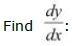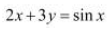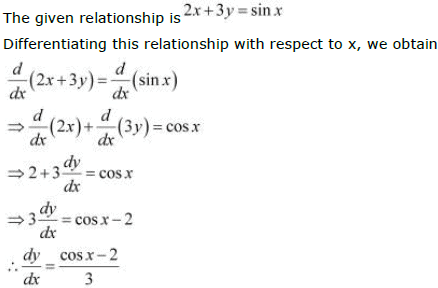Question 2: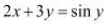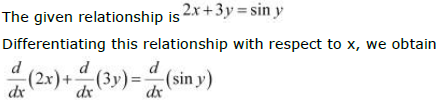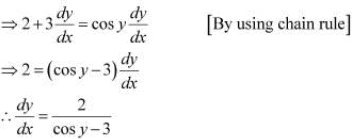Question 3: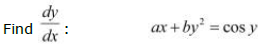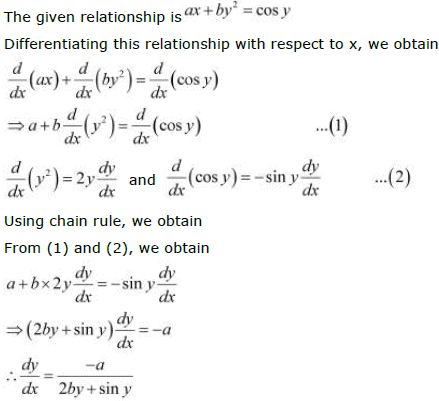Question 4: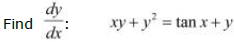The given relationship is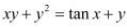Differentiating this relationship with respect to x, we obtain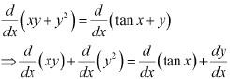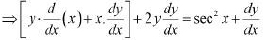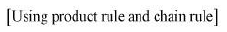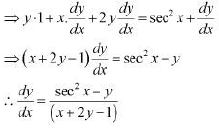Question 5:

Find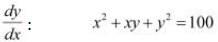The given relationship is x2 +xy +y2 = 100

Differentiating this relationship with respect to x, we obtain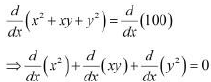[Derivative of constant function is 0]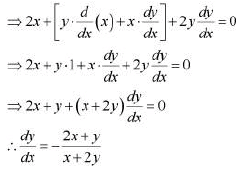Question 6: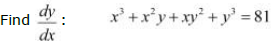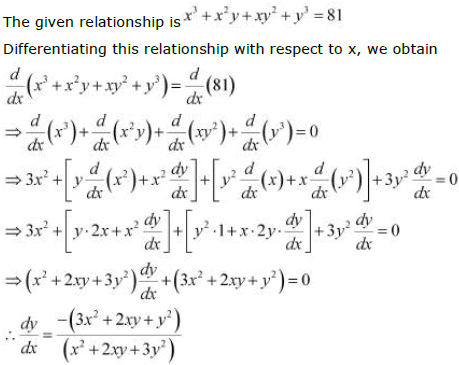Question 7: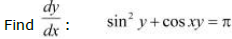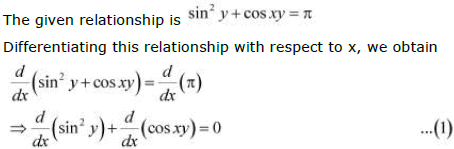Using chain rule, we obtain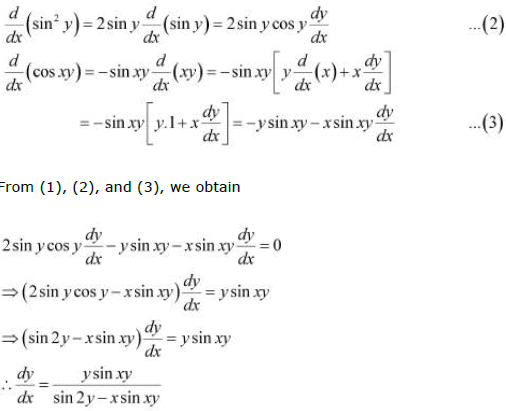Question 8:

Find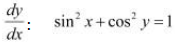The given relationship is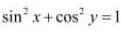Differentiating this relationship with respect to x, we obtain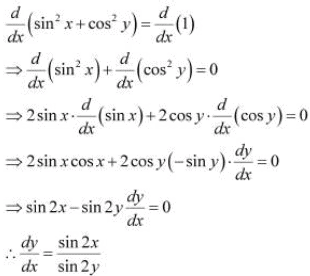Question 9:

Find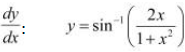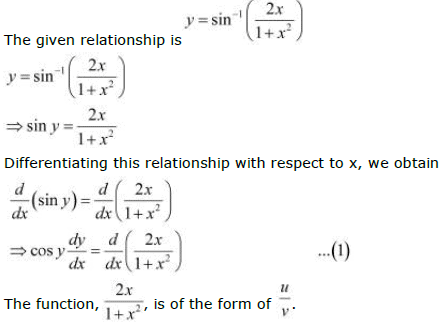Therefore, by quotient rule, we obtain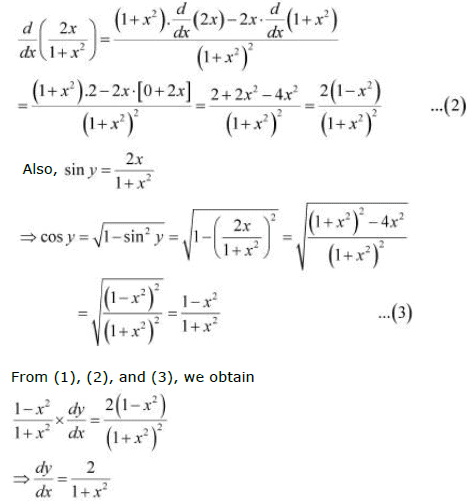Question 10: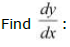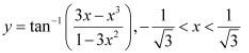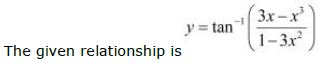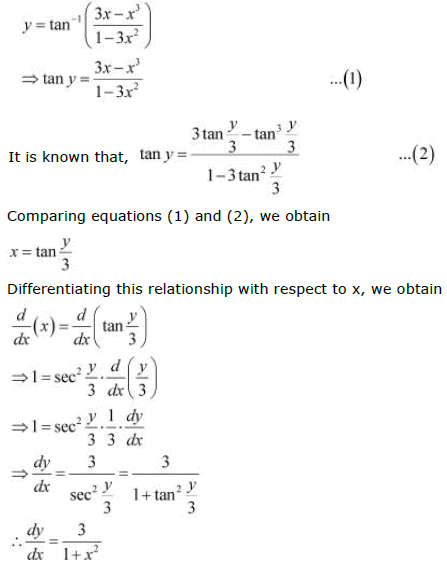Question 11: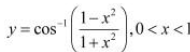The given relationship is,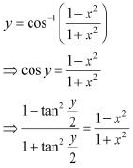On comparing L.H.S. and R.H.S. of the above relationship, we obtain  tany/2 = x

Differentiating this relationship with respect to x, we obtain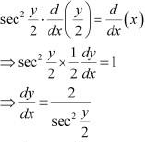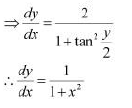Question 12: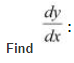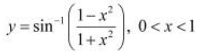The given relationship is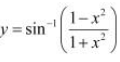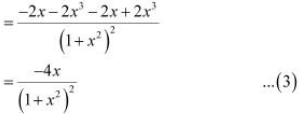From (1), (2), and (3), we obtain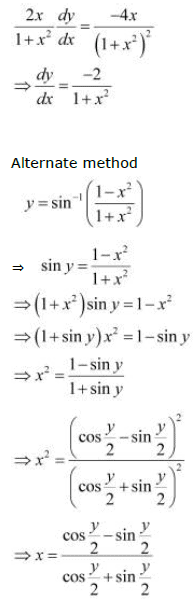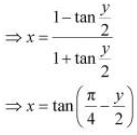Differentiating this relationship with respect to x, we obtain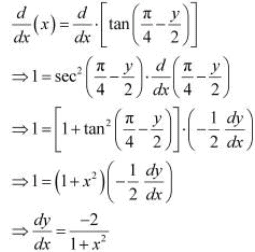Question 13: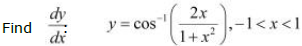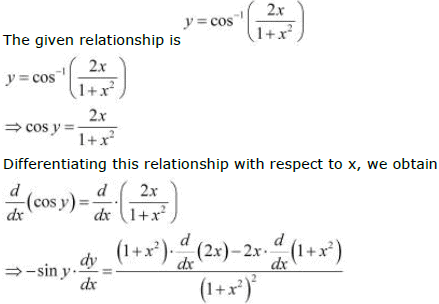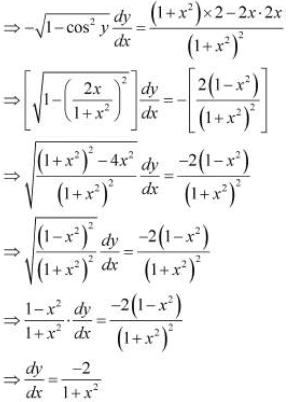Question 14:

Find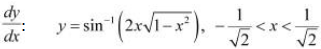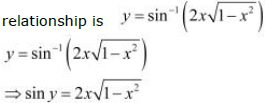Differentiating this relationship with respect to x, we obtain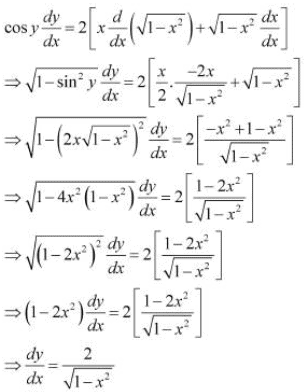Question 15:

Find dy/dx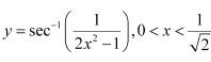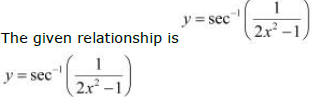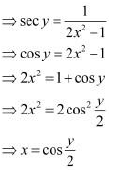Differentiating this relationship with respect to x, we obtain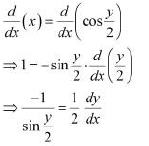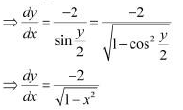Offer running on EduRev: Apply code STAYHOME200 to get INR 200 off on our premium plan EduRev Infinity!

## Mathematics (Maths) Class 12

209 videos|222 docs|124 tests

,

,

,

,

,

,

,

,

,

,

,

,

,

,

,

,

,

,

,

,

,

,

,

,

;